Maths-
General
Easy

Question

# Sides of triangle are 100 m, 120 m and 140 m. Find its areaHint:

## The correct answer is: 2.44

### It is given that a = 100 , b = 120 and c = 140Using Heron’s formulaArea of triangle =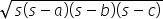where s =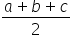s =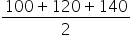= 180Area of triangle=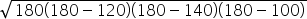=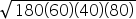= 100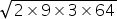= 2400 cm2= 5878.76 cm2           (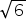= 2.44)#### With Turito Foundation.#### Get an Expert Advice From Turito.